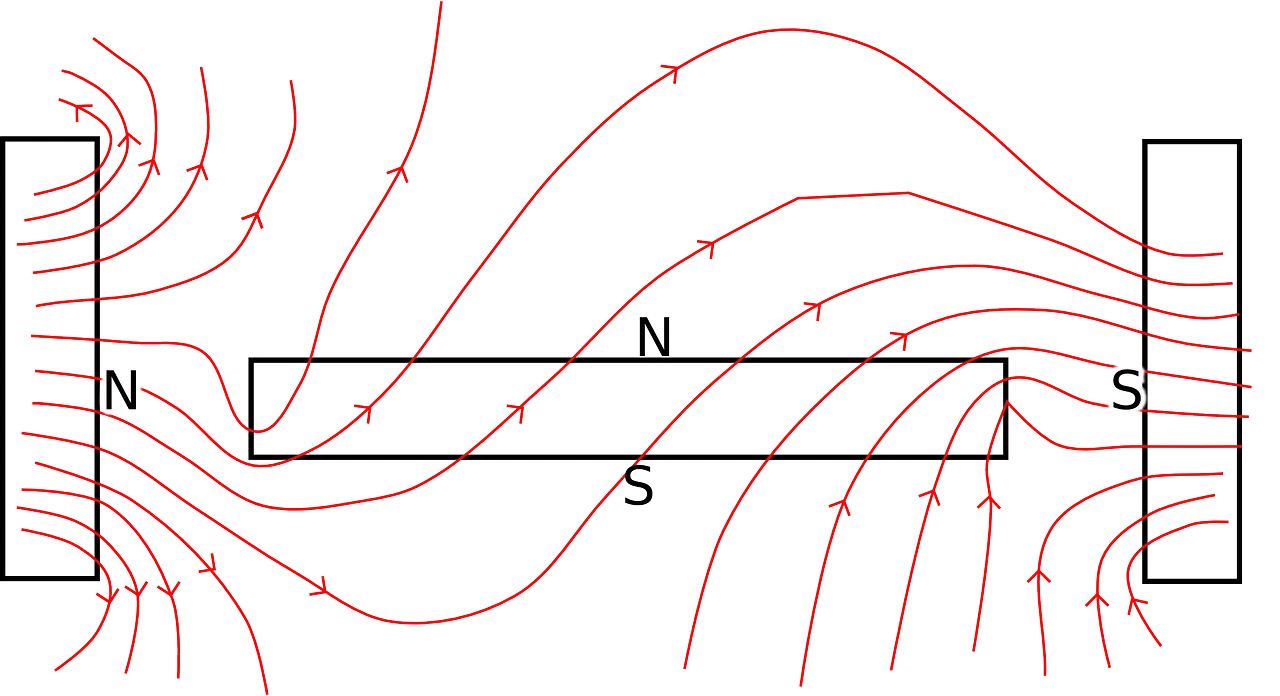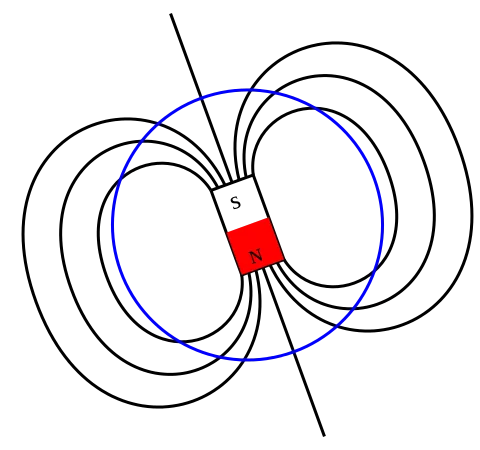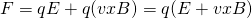# Physics - Electromagnetism - Magnetic fields## Introduction

Hello it's a me again Drifter Programming! Today we continue with Electromagnetism to get into Magnetic fields. What we covered 'till now was mostly the "Electricity" part of Electromagnetism. With this post we finally get into Magnetism too. The two main things that I will cover is what Magnetism is and we will also get into Magnetic fields. So, without further do, let's get straight into it!

## Magnetism

First things first magnets and magnetism is called like that because of the region in Asia Minor called Magnesia. The name comes from the greek word: "μαγνήτης" (magnitis) that comes from the region's name: "Μαγνησία" (Magnisia), which now is a part of western Turkey. The mineral material Fe3O4 which is called magnetite also comes from the same greek word.

Magnetic objects or magnets are those that attract or pull on metals. For example rubbing a piece of steel with a strong magnet makes that piece also a magnet. When that happens we say that the steel has become magnetized. Of course not all materials can be magnetized and so not all materials are attracted by magnets. Magnets also attract/pull each other if they face in opposite directions. With direction I mean that all magnets have two ends which are called poles and that we call north and south pole. Opposite poles (north and south) attract each other, whilst the same poles (north and north OR south and south) repel each other. Can you see the similarity with electric charges? Electricity and Magnetism are very close related!

Rule: Opposite poles attract, whilst like poles repel.

So, why do we call them north and south pole? Well, it's simple, the Earth is a giant magnet with two poles. A compass (tiny magnet that moves freely) is attracted by the Earth's magnetic north pole and so always points in that direction, which is why we call them like that. Of course the magnetic poles of the earth are not in the same spot as the geographical poles and are also opposite (the Earth's geographical north pole is near the magnetic south pole). The axis lines of each are the so called magnetic and geographical meridians. There is a angle between them which is the so called magnetic declination that is also reducing right now. The magnetic field of the earth also reverses itself every million (the poles switch). The magnetic field of the Earth is very important cause it protects the Earth from solar wind, which is a stream of charged particles emmited from our Sun. These particles are trapped in the Earth's magnetic field and are responsible for the Aurora seen in the sky around the poles.The three basic magnetic elements are:

• Iron (Fe)
• Nickel (Ni)
• Cobalt (Co)

Electric motors, generators, recycling facilities, hard drives, tape recorders and high-speed leviation trains are only some of the applications of magnets and magnetic fields. Magnetism studies the properties of those magnets, the effect of the magnetic force on moving charges and currents and also the creation of magnetic fields by currents. There goes a lot of study into them, because of such a wide range of applications.

## Magnetic Fields

The same way as electric charge produced an electric field, magnets create magnetic fields. A magnet applies a force called Magnetic Force to objects (like charges) that are attracted or repelled by magnets. The force applied to a charge depends on the charge, velocity and angle to the field. The particles/charges are deflected by that force and don't change their speed. The magnetic field which is sometimes called magnetic induction or magnetic flux density is symbolized by B. The actual "power" of magnetic fields is measured in units of Tesla (T). The magnetic force exerted on particles in a field is also called Lorentz force. The motion of electrically charged particles gives rise to magnetism. The force acting on an electrically charged particle in a magnetic field depends on the magnitude of the charge, the velocity of the particle, and the strength of the magnetic field.

A magnetic field looks like this:Image source:Exactly the same way as with electric field lines, the magnetic force is applied in the direction of the magnetic field lines. You can also clearly see the similarity to electric dipoles that are build up of '+' and '-' that can be compared to the north and south pole. The field lines are noted to go from the north to the south pole and so the direction of the magnetic field is north to south pole. We will cover more about them next time...

When being inside of an magnetic and electric field the total force applied to a charged particle is:which is Lorentz's force law. Of course vxB represents the cross-product of the velocity vecotr with the magnetic field vector. The direction of the electric force depends on the charge, but will always be in the same "axis" as the electric field. On the other hand the magnetic force is given by the right hand rule. This mnemonic law of visualizing the direction of the magnetic force is very useful. When having a positive charge we can do either of the following:

1. Curl our fingers as if rotating vector v into vector B. The thumb shows us the direction of the force.
2. Point thumb into the direction of the velocity, fingers in the field direction. The palm now shows us the direciton of the force.

When having negative charges the force goes in the opposite direction. This means that we could also just use the left hand and do the same. For a visual representation I suggest you to read: http://hyperphysics.phy-astr.gsu.edu/hbase/magnetic/magfor.html#c3

## REFERENCES:

Mathematical equations that I had in this post where drawn using quicklatex

### Electric fields:

Getting into Electromagnetism -> electromagnetim, electric charge, conductors, insulators, quantization

Coulomb's law with examples -> Coulomb's law, superposition principle, Coulomb constant, how to solve problems, examples

Electric fields and field lines -> Electric fields, Solving problems around Electric fields and field lines

Electric dipoles -> Electric dipole, torque, potential and field

Electric charge and field Exercises -> examples in electric charges and fields

### Electric flux:

Electric flux and Gauss's law -> Electric flux, Gauss's law

Applications of Gauss's law (part 1) -> applying Gauss's law, Gauss applications

Applications of Gauss's law (part 2) -> more Gauss applications

Electric flux exercises -> examples in electric flux and Gauss's law

### Electric potential:

Electric potential energy -> explanation of work-energy, electric potential energy

Calculating electric potentials -> more stuff about potential energy, potential, calculating potentials

Millikan's Oil Drop Experiment -> Millikan's experiment, electronvolt

Cathode ray tubes explained using electric potential -> cathode ray tube explanation

Electric potential exercises (part 1) -> applications of potential

Electric potential exercises (part 2) -> applications of potential gradient, advanced examples

### Capacitance:

Capacitors (Condensers) and Capacitance -> Capacitors, capacitance, calculating capacitance

How to solve problems around Capacitors -> combination, solving problems, simple example

Electric field energy and density -> Electric field energy, energy density

Dielectric materials -> Dielectrics, dielectric constant, permittivity and strength, how to solve problems

Electric capacitance exercises -> examples in capacitance, energy density and dielectrics

### Current, resistance and EMF:

Electric current -> Electric current, current density

Electrical resistivity and conductivity -> Electrical resistivity, conductivity, thermal coefficient of resistivity, hyperconductivity

Electric resistance -> Resistance, temperature, resistors

Electromotive Force (EMF) and Internal resistance -> Electromotive force, internal resistance

Power and Wattage of Electronic Circuits -> Power in general, power/wattage of electronic circuits

Electric current, resistance and emf exercises -> exampes in all those topics

### Direct current (DC) circuits:

Resistor Combinations -> Resistor combinations, how to solve problems

Kirchhoff's laws with applications -> Kirchhoff's laws, how to solve problems, applications

Electrical measuring instruments -> what are they?, types list, getting into some of them, an application

Electronic circuits with resistors and capacitors (R-C) -> R-C Circuit, charging, time constant, discharging, how to apply

RC circuit exercises -> examples in Kirchhoff, charging, discharging capacitor with/without internal resistance

And this is actually it for today's post!

Next time we will get into Magnetic field lines and Gauss's law of Magnetism.

Bye bye!

H2
H3
H4
3 columns
2 columns
1 column